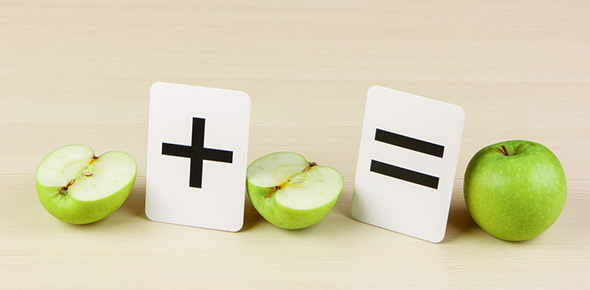# Fractions

11 Questions | Total Attempts: 164SettingsBasic knowledge of fractions, how to order them, finding the LCM, adding and subtracting fractions, variables, and expressions.

Related Topics
• 1.
Make a factor tree for 8.
• 2.
Write "the sum of X plus 3" as a mathematical expression.
• A.

X = 3

• B.

X + 3

• C.

3 = X + 3

• 3.
Write "5 less than a number" as a mathematical expression.
• A.

X - 5

• B.

5 - X

• C.

X = 5 - X

• 4.
List all possible factors of 24.
• 5.
Which fraction below is equal to 1/2 ?
• A.

1/4

• B.

2/6

• C.

2/4

• 6.
Indicate whether the fraction is greater than, less than or equal to.2/7 ____  4/7
• A.

Less than

• B.

Greater than

• C.

Equal to

• 7.
Indicate whether the fraction is less than, greater, than, or equal to.4/5 _____  9/8
• A.

Equal to

• B.

Greater than

• C.

Less than

• 8.
Solve the fraction problem and write in simplest form.2/4  + 2/4 =
• A.

4/8

• B.

1

• C.

2/8

• 9.
Solve the fraction problem and write in simplest form.1/3 + 1/4 = _____
• A.

7/12

• B.

2/7

• C.

2/12

• 10.
Solve the fraction problem and write in simplest form.4/6 - 2/6 = _____
• A.

2/6

• B.

1/3

• C.

1

• 11.
Solve the fraction problem and write in simplest form.1/2 - 1/3 = _____
• A.

2/3

• B.

2/6

• C.

1/6